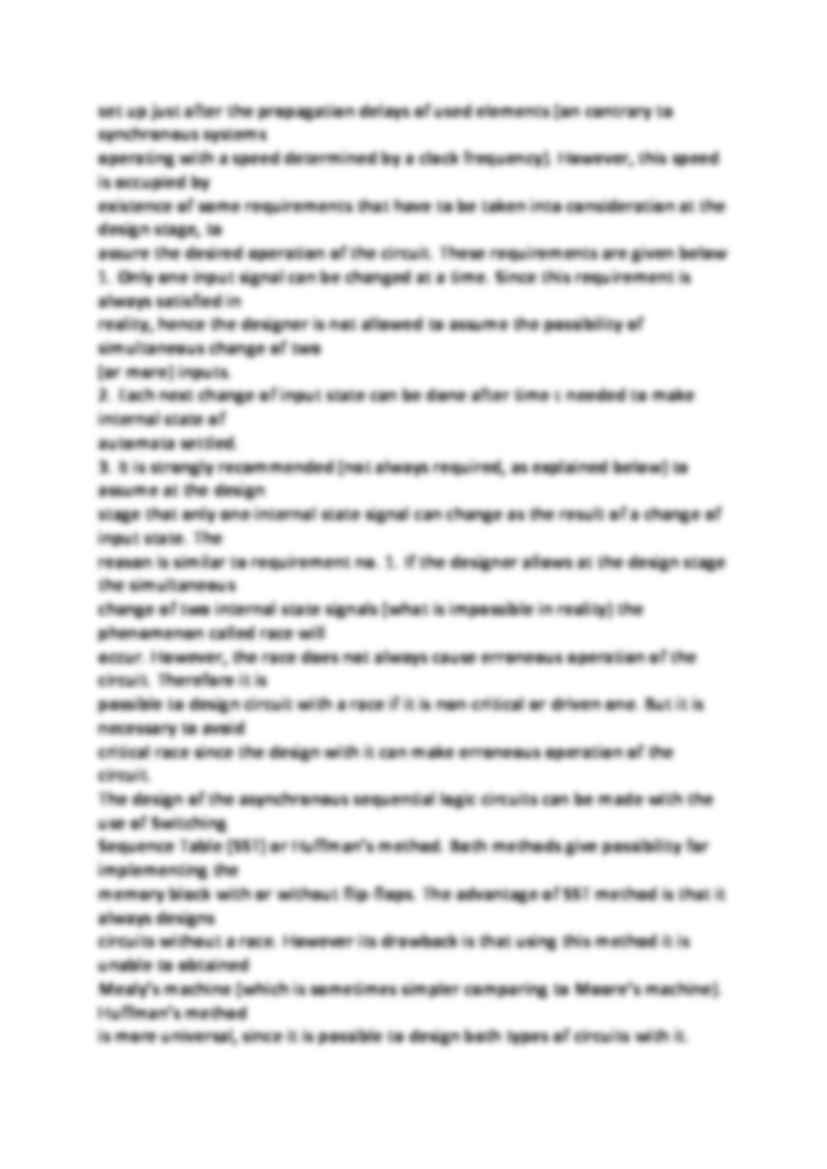# Asynchronous sequential logic circuits

Nasza ocena:

Pobrań: 42
Wyświetleń: 826
Komentarze: 0

### Pobierz ten dokument za darmo

Podgląd dokumentu## Fragment notatki:

Asynchronous sequential logic circuits All switching circuits belong to one of two classes: combinational or sequential. On contrary to
combinational logic circuits, the operation of sequential ones is dependent not only on the present
state of external inputs, but also on the state of these inputs in past. Therefore, sequential logic
circuits are sometimes referred to as circuits with memory. The memory block is formed by some
additional signals looped backward from the output to the input of the circuit. These signals are
referred to as signals of internal state of the circuit. By this backward loop, the dependence on
previous values of inputs is implemented as dependence on the current internal state of the
machine.
The sequential switching circuits are asynchronous or synchronous. Asynchronous ones operate
at time events defined by changes of inputs (on contrary to synchronous circuits operating at time
events defined by additional control signal called clock). Asynchronous sequential logic circuits
can be further divided into two subclasses: fundamental mode (or static), and pulse mode (or
dynamic) circuits. Fundamental mode asynchronous switching circuits operate according to
voltage levels of inputs, while pulse mode ones operate according to changes in voltage levels.
Since dynamic asynchronous sequential logic circuit are very sensitive on error condition like
spikes, they are used very rarely and will not be discussed here.
Let us denote by x the input vector and by Z the output vector. Furthermore, let q and Q denote
vectors of internal state of the machine at present and at next time event respectively. Then the
operation of asynchronous sequential circuit can be formally assigned as:
if Mealy's machine is concerned, or as for Moore's machine.
Asynchronous sequential logic circuits operate faster than synchronous ones, as the outputs are
set up just after the propagation delays of used elements (on contrary to synchronous systems
operating with a speed determined by a clock frequency). However, this speed is occupied by
existence of some requirements that have to be taken into consideration at the design stage, to
assure the desired operation of the circuit. These requirements are given below
1. Only one input signal can be changed at a time. Since this requirement is always satisfied in
reality, hence the designer is not allowed to assume the possibility of simultaneous change of two
(or more) inputs.
2. Each next change of input state can be done after time τ needed to make internal state of
automata settled.
3. It is strongly recommended (not always required, as explained below) to assume at the design
... zobacz całą notatkę

## Komentarze użytkowników (0)

Zaloguj się, aby dodać komentarz